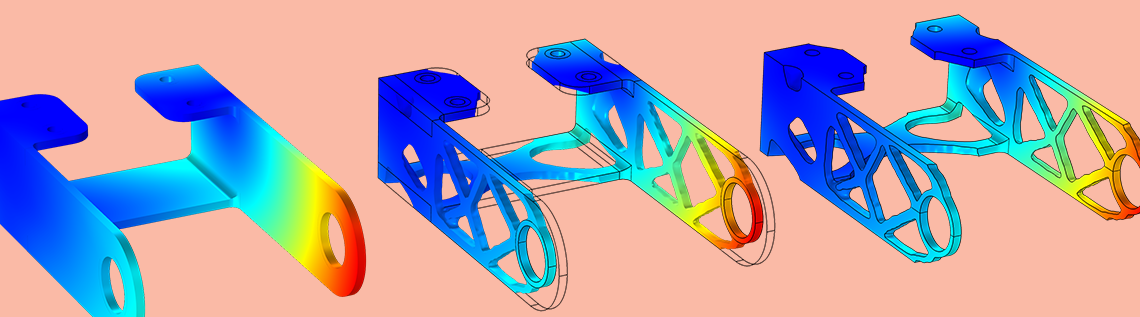# Performing Topology Optimization with the Density Method

January 4, 2019

Engineers are given significant freedom in their pursuit of lightweight structural components in airplanes and space applications, so it makes sense to use methods that can exploit this freedom, making topology optimization a popular choice in the early design phase. This method often requires regularization and special interpolation functions to get meaningful designs, which can be a nuisance to both new and experienced simulation users. To simplify the solution of topology optimization problems, the COMSOL® software contains a density topology feature.

### About the Density Method for Topology Optimization

As the name suggests, topology optimization is a method that has the ability to come up with new and better topologies for an engineering structure given an objective function and set of constraints. The method comes up with these new topologies by introducing a set of design variables that describe the presence, or absence, of material within the design space. These variables are defined either within every element of the mesh or on every node point of the mesh. Changing these design variables thus becomes analogous to changing the topology. This means that holes in the structure can appear, disappear, and merge as well as that boundaries can take on arbitrary shapes. In addition, the control parameters are somewhat automatically defined and tied to the discretization.

As of COMSOL Multiphysics® software version 5.4, the add-on Optimization Module includes a density topology feature to improve the usability of topology optimization. The feature is designed to be used as a density method (Ref. 3), meaning that the control parameters change a material parameter through an interpolation function. Interpolation functions for solid and fluid mechanics are built into the feature and used in example models throughout the Application Library in COMSOL Multiphysics.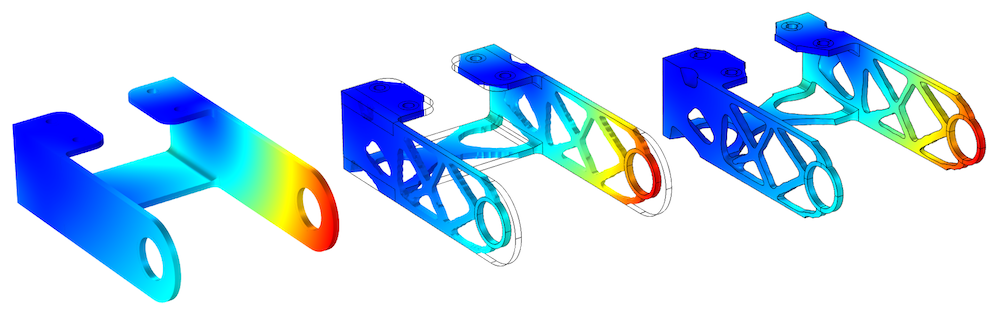A bracket geometry is topology optimized, leaving only 50% of the material, which contributes the most to the stiffness.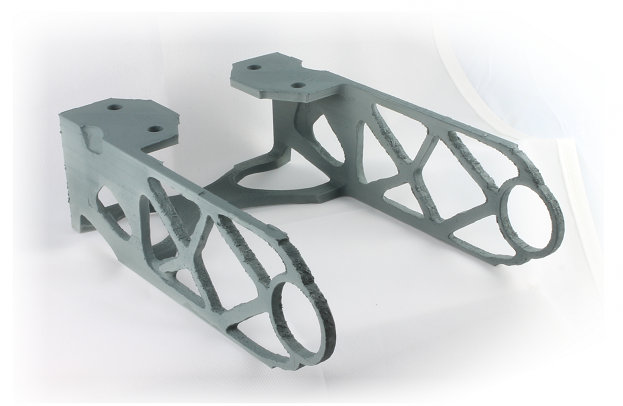The printed bracket geometry.

The density method involves the definition of a control variable field, \theta_c, which is bounded between 0 and 1. In solid mechanics, \theta_c=1 corresponds to the material from which the structure is to be built, while \theta_c=0 corresponds to a very soft material. By default, the void Young’s modulus is 0.1% of the solid Young’s modulus. In fluid mechanics, convention dictates that \theta_c=1 corresponds to fluid, while \theta_c=0 is a (slightly) permeable material with an inverse permeability factor, \alpha; i.e., a damping term is added to the Navier-Stokes equation:

\rho \frac{D\mathbf{v}}{Dt} = -\mathbf{\nabla}p+\eta\nabla^2\mathbf{v}-\alpha(\theta_c)\mathbf{v}

The damping term is 0 in fluid domains, while a large value is used in solid domains. These different values give a good approximation of the no-slip boundary condition on the interface between the domains.

### An Introduction to the Density Model Feature

The Density Model feature supports regularization via a Helmholtz equation (Ref. 1). This introduces a minimum length scale using the filter radius, R_\mathrm{min}:

\theta_f = R_\mathrm{min}^2\mathbf{\nabla}^2\theta_f + \theta_c.

Here, \theta_c is the raw control variable, which is modified by the optimizer, and \theta_f is the filtered variable. The mesh edge size is the default value for the filter radius. While this works well in terms of regularizing the optimization problem, it’s important to set a fixed length (larger than the mesh edge size) to get mesh-independent results.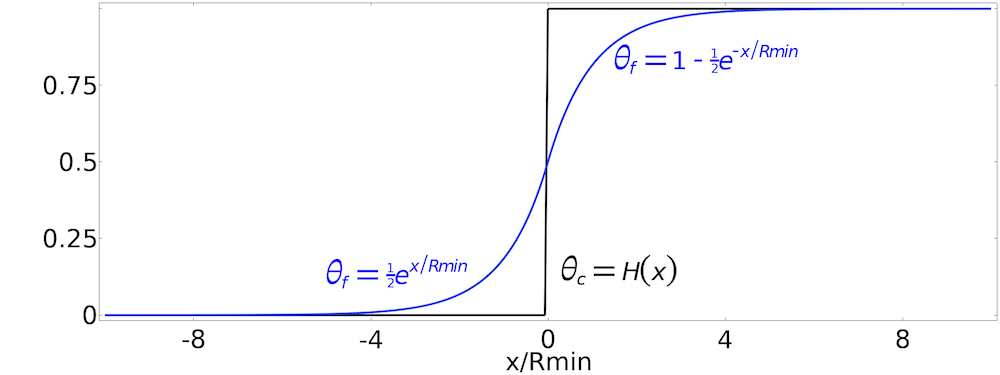Top: The equation for the Helmholtz filter can be solved analytically for a 1D Heaviside function. Bottom: This plot is taken from the MBB beam optimization model. It shows the raw control variables to the left and the filtered version to the right.

The Helmholtz filter gives rise to significant grayscale, which does not have a clear physical interpretation. The grayscale can be reduced by applying a smooth step function in what is referred to as projection in topology optimization. Projection reduces grayscale, but it also makes it more difficult for the optimizer to converge. The density topology feature supports projection based on the hyperbolic tangent function, and the amount of projection can be controlled with the projection steepness, \beta.

\theta = \frac{\tanh(\beta(\theta_f-\theta_{\beta}))+\tanh(\beta\theta_{\beta})}{\tanh(\beta(1-\theta_{\beta}))+\tanh(\beta\theta_{\beta})}

Here, \theta_{\beta} is the projection point.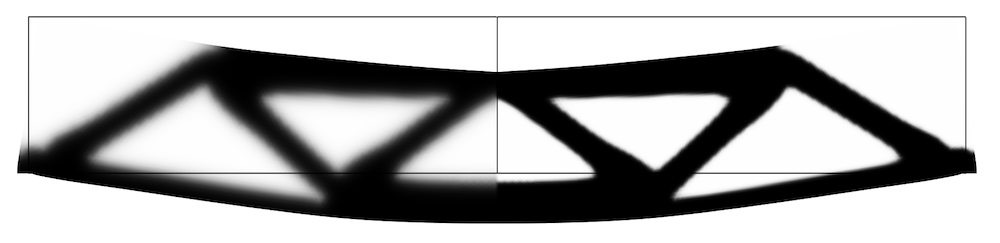Plot showing the filtered field to the left and the projected field to the right.

Projection makes it possible to avoid grayscale, but grayscale can still appear if the optimization problem favors it. If the same interpolation function is used for the mass and the stiffness, grayscale is optimal in volume-constrained minimum compliance problems. It is thus common to use interpolation functions that cause intermediate values to be associated with little stiffness relative to their cost (compared to the fully solid value). You can think of this as a penalization of intermediate values for the material volume factor, and the Density Model interface (shown below) supports two such interpolation schemes for solid mechanics: solid isotropic material with penalization (SIMP) and rational approximation of material properties method (RAMP) interpolation. Darcy interpolation is provided for fluid mechanics. The interpolated variable is called the penalized material volume factor, \theta_p, and is used for interpolating the material parameters, e.g., for SIMP interpolation, the p_\textsc{simp} exponent can be increased to reduce the stiffness of intermediate values, so that grayscale becomes less favorable.

\begin{align}
\theta_p %26= \theta_\mathrm{min}(1-\theta_\mathrm{min})\theta^{p_\textsc{simp}}\\
E_p %26= E\theta_p
\end{align}

Here, E is the Young’s modulus of the solid material and E_p is the penalized Young’s modulus to be used throughout all optimized domains.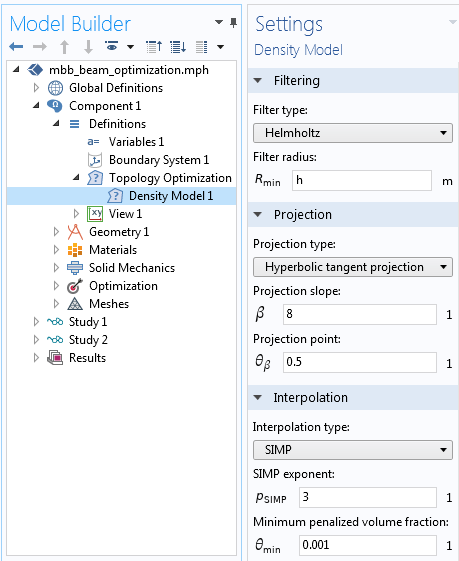The Density Model feature is available under Topology Optimization in Component > Definitions. The mesh edge length is taken as the default filter radius and it works well, but it has to be replaced with a fixed value in order to produce mesh-independent results.

The penalized Young’s modulus can be defined as a domain variable, or (as in the case of the bracket model) it can be defined directly in the materials.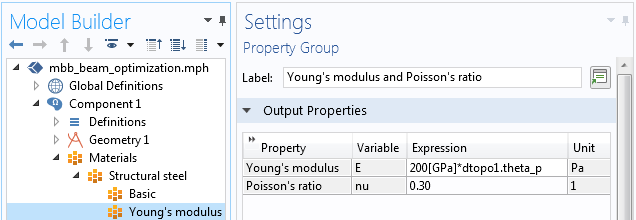Topology optimization with the density method involves varying the Young’s modulus spatially. In this case, it is achieved by going to the material properties and multiplying the solid Young’s modulus with the penalized material volume factor, dtopo1.theta_p.

In summary, the density topology feature adds four variables. The filtered material volume factor is defined implicitly using a dependent variable.

Symbol Description Equation
\theta_c Control material volume factor 0\leq\theta_c\leq1
\theta_f Filtered material volume factor \theta_f = R_\mathrm{min}^2\mathbf{\nabla}^2\theta_f + \theta_c
\theta Material volume factor \theta = \frac{\tanh(\beta(\theta_f-\theta_{\beta}))+\tanh(\beta\theta_{\beta})}{\tanh(\beta(1-\theta_{\beta}))+\tanh(\beta\theta_{\beta})}
\theta_p Penalized material volume factor \theta_p = \theta_\mathrm{min}+(1-\theta_\mathrm{min})\theta^{p_\textsc{simp}} or \theta_p = \frac{q_\mathrm{Darcy}(1-\theta)}{q_\mathrm{Darcy}+\theta}

When the filtering is disabled, the filtered variable becomes undefined and the projection instead uses the control material volume factor directly. If the projection is disabled, the material volume factor still exists, but it becomes identical to the projection input.

### Applying Continuation to Avoid Local Minima

When the topology is not too complicated, the default values of the density topology feature work well. This is the case for the MBB beam optimization and topology optimized hook models. If the optimal design is more complicated (such as for the bracket example shown at the top of this post), there might be many local minima. To avoid these minima, you can use continuation in the SIMP exponent and the projection slope. This can be achieved by modifying the initial value expression in the density topology feature and adding a Parametric Sweep feature, as shown below. As a result, the solver ramps over the specified parameters, using the optimum from the previous case as the initial value for the next optimization step. That is, it starts with a small SIMP exponent and projection slope and then continues to higher values.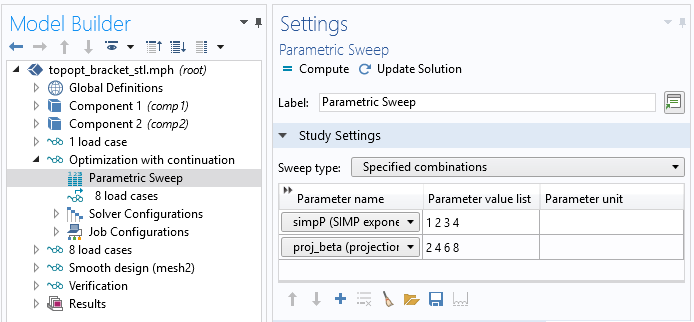It is possible to apply continuation by combining a parametric sweep with a study reference. See the Bracket — Topology Optimization tutorial model for details.

### Objectives and Constraints in Topology Optimization

If the geometry is optimized for a single load case (as shown below to the left), the resulting design will be optimal with respect to that load case. This can seem obvious, but often designers make assumptions about symmetries and the design topology. Unless these assumptions are formalized as constraints, they will not be respected. Therefore, the design shown to the right below uses eight load cases (two load groups times four constraint groups).

Left: The bracket geometry is optimized for a single load case, resulting in an asymmetric design with two loosely connected halves. Right: The bracket geometry with eight load cases.

Designers often have several objectives that need to be weighted. To make an informed decision about these objectives, a designer can trace the Pareto optimal front using several optimizations with different weights.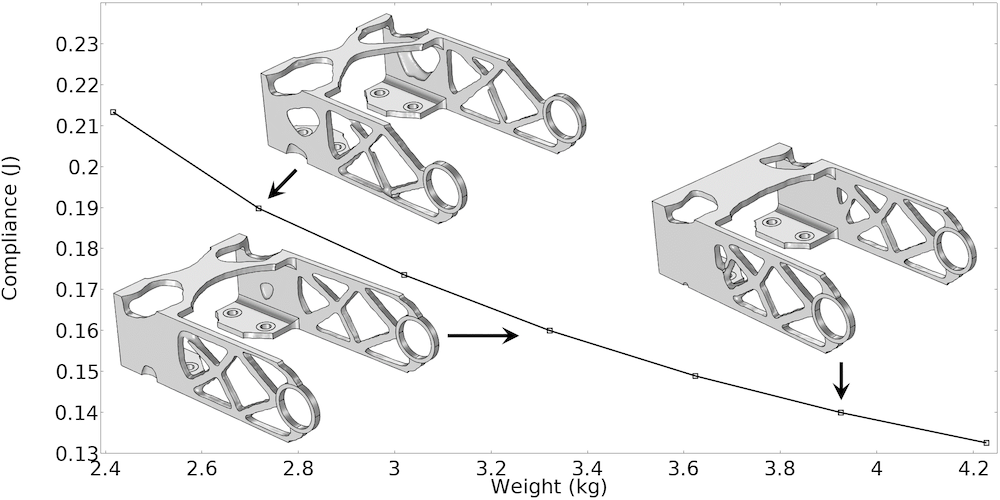The Pareto optimal front for the bracket geometry can be traced by varying the weight in a parametric sweep.

Animation of the topology optimized bracket. (Download the glTF™ file from the Application Gallery in GLB-file format to rotate the geometry yourself.)

### Exporting and Importing Topology Optimization Results

It is possible to analyze the result of a topology optimized design with respect to stress concentration and buckling without remeshing. However, if you want to be completely sure that the void phase does not play a role, you can eliminate it by exporting and importing the resulting design, as shown below. The details of this procedure are discussed in a previous blog post.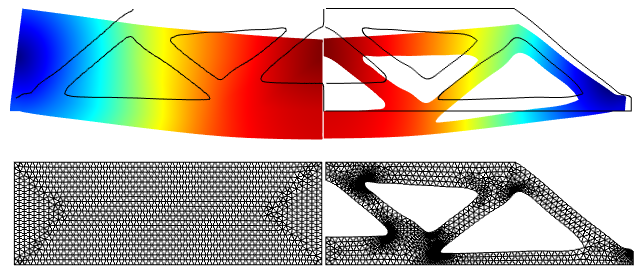The contour (left) for the topology optimized MBB beam design is exported and imported as an interpolation curve (right).

### Next Steps

To learn more about the built-in tools and features for solving optimization problems, check out the Optimization Module product page by clicking the button below.

#### Further Resources

• Try using the density feature for topology optimization with these example models:
• Read more about topology optimization on the COMSOL Blog:
1. B.S. Lazarov and O. Sigmund, “Filters in topology optimization based on Helmholtz‐type differential equations,” International Journal for Numerical Methods in Engineering, vol. 86, no. 6, pp. 765–781, 2011.
2. F. Wang, B.S. Lazarov, and O. Sigmund, “On projection methods, convergence and robust formulations in topology optimization,” Structural and Multidisciplinary Optimization, vol. 43, pp. 767–784, 2011.
3. M.P. Bendsøe, “Optimal shape design as a material distribution problem,” Structural Optimization, vol. 1, pp. 193–202, 1989.

glTF and the glTF logo are trademarks of the Khronos Group Inc.

#### Categories